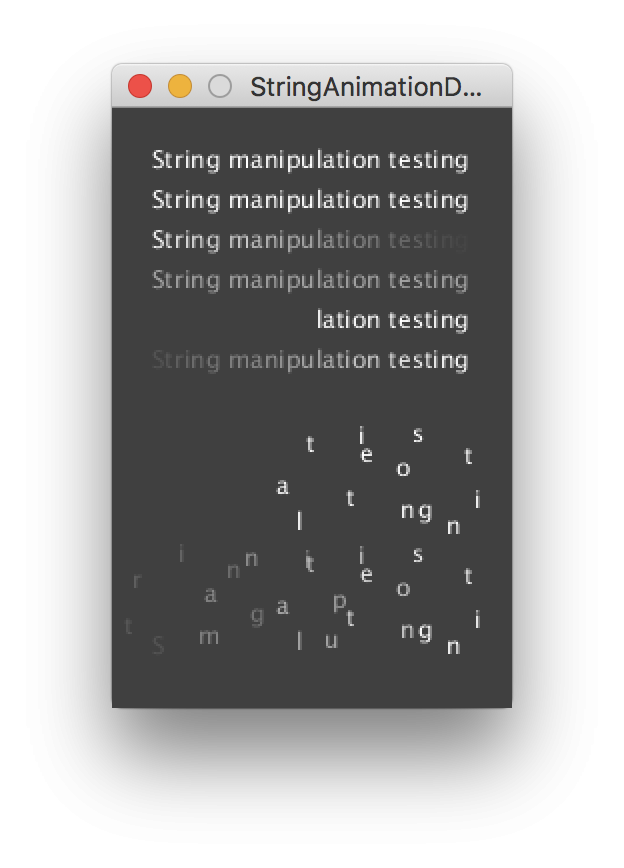#### Howdy, Stranger!

We are about to switch to a new forum software. Until then we have removed the registration on this forum.

# How to make ascending and descending list of numbers, based on time.

edited April 2018

I am trying to create a boomerang (instagram) type effect for 3D text, where the text rotates from one orientation then rotates the opposite direction but is smooth and does not jump. This rotation should continue in an infinite loop (example for video shown here ). The closest I can get is

``````p.draw = function() {

if (mouseIsPressed) {
p.background('#c3f2cf');

p.push();
p.translate(-60, 50, 35);
if (second() % 2 == 0) {
setInterval(function() {i = i + 1}, 500);
} else {
setInterval(function() {i = i - 1}, 500);
}
p.rotateX(i);
p.texture(img2);
p.scale(0.7);
p.model(dearlove);
p.pop();
}
else {
p.background(255);
}
}
``````

I am using instance mode here. Essentially I am trying to make an ascending and descending list of numbers that is passed to the p.rotateX for the p.model(dearlove). But the numbers should ascend to one value, then descend FROM that value. I can almost get the effect I want by

``````p.draw = function() {

if (second()%2 == 0) {
i = 0.03;
} else {
i = -0.03
}

p.push();
p.translate(-60, 50, 35);
p.rotateX(p.frameCount * i);
p.texture(img2);
p.scale(0.7);
p.model(dearlove);
p.pop();

}
``````

but the 3D text jumps orientations when switching the direction of rotation. I'm sure I'm over thinking this. Cannot figure out how to get around the lack of a sleep() function in javascript. Let me know if further clarification is needed. Thanks.

Tagged:

• One way of approaching this kind of problem is:

1. produce a function that maps animated text locations to 0-1 input.
2. use `sin()` to generate input, and `map()` (-1,1) to (0,1) to bounce back and forth.

For previous example, see:• edited April 2018

Thanks for the quick response, I have attempted a solution using what you recommended which looked something like

``````    var PH8 = function ( p ) {
var myCanvas;
var img;
var img2;

}

p.setup = function() {
myCanvas = p.createCanvas(canvasWidth, canvasHeight, p.WEBGL);
}

p.windowResized = function() {
p.resizeCanvas(canvasWidth, canvasHeight);
}

p.draw = function() {
if (mouseIsPressed) {
p.background('#c3f2cf');

p.push();
p.translate(micLevel*1000+200, micLevel*300+100, 100);
p.normalMaterial();
p.rotateX(3.14159);
p.rotateY(0.78539+ p.frameCount*0.05);
p.scale(0.5);
p.model(comma);
p.pop();

p.push();
p.translate(-60, 50, 35);
var preMap = sin(p.frameCount);
var postMap = p.map(preMap, -1, 1, -3.14, 0);
console.log(postMap);

p.rotateX(postMap);
p.texture(img2);
p.scale(0.9);
p.model(dearlove);
p.pop();

p.push();
p.translate(-55, -80, 25);
p.rotateX(p.frameCount * 0.19);
p.rotateY(0);
p.texture(img2);
p.scale(9);
p.model(icantthink);
p.pop();
// p.fill(r, g, b);
}
else {
p.background(255);
}
}
}
myp5 = new p5(PH8, 'container8');
``````

but the output from the sine function is still very jumpy. I have attempted another solution that looks like the following(I have removed extraneous lines of code)

``````    var PH8 = function ( p ) {
var myCanvas;
var m;

p.countUp = function() {
m += 1;
}

p.countDown = function() {
m -= 1;
}

p.setup = function() {
myCanvas = p.createCanvas(canvasWidth, canvasHeight, p.WEBGL);

m = 0;

setInterval(function() {
if (second() % 2 == 0) {
clearInterval(down);
var up = setInterval(p.countUp, 100);
} else {
clearInterval(up);
var down = setInterval(p.countDown, 100);
}
}, 1000);
}

p.draw = function() {
if (mouseIsPressed) {
p.background('#c3f2cf');

console.log("m = " + m);
}
else {
p.background(255);
}
}
}
`myp5 = new p5(PH8, 'container8');`
``````

and this almost works, as the numbers do oscillate up and down, but the sequence of numbers approaches infinity rather than forever oscillating. Any thoughts? Thanks.

• Just got this to work! Needed to define the variable for the setInterval outside of the if statement. Thanks for your help!

• Just now noticing that this works around 80% of the time. Occasionally negativie numbers win out, and the model rotates only one direction, rather than bouncing. Currently am playing with the timing of the setIntervals, to see if this has an effect.

• edited April 2018 Answer ✓

Notice that it seems `image()` is not yet (properly) implemented in p5.js when using the WebGJ renderer. See https: //github.com/processing/p5.js/issues/1507

Kf

``````// Boomerange effect - Proof of concept
// REFERENCE: https:// forum.processing.org/two/discussion/27681/how-to-make-ascending-and-descending-list-of-numbers-based-on-time#latest
//
//NOTICE: WebGL doesn't seem to fully implement Processing functions such as image(). More at https://github.com/processing/p5.js/issues/1507

var PH8 = function ( p ) {
var myCanvas;
var m;
var upFlag;
var currentTimer;
var pg;

var img;
var img2;
var coma, dearlove, icantthink;

}

p.setup = function() {
myCanvas = p.createCanvas(600, 400, p.P2D);

pg = p.createGraphics(p.width-100, p.height-200,p.WEBGL);
p.imageMode(p.CENTER,p.CENTER);
m = 0;
upFlag=1;
img.resize(p.width/4,0);

setInterval(function() {
clearInterval(currentTimer);
if (upFlag==0) {
currentTimer = setInterval(p.countUp, 100);
upFlag=1;
} else {
currentTimer = setInterval(p.countDown, 100);
upFlag=0;
}
},1000);

}// Setup

p.draw = function() {
p.background('#c3f2cf');
//console.log("m = " + m);
p.translate(p.width/2,p.height/2);
p.rotate(m/10.0);
if (p.mouseIsPressed) {
p.image(img,0,0);

} else {

//pg.background(190,255,190);
//pg.push();
//pg.translate(p.width/2,p.height/2, 100);
//pg.normalMaterial();
//pg.rotateX(3.14159);
//pg.rotateY(0.78539+ p.frameCount*0.05);
//pg.scale(0.5);
//pg.fill(150);
//pg.stroke(0,150,25);

//var pg2 = p.createGraphics(256,256);
//pg.box(p.witdth/2,50,50);
//pg2.text('hello world!', 50, 50);
//pg.texture(pg2);
//pg.pop();
//p.image(pg,50,0);
p.image(img2,0,0);
}
}

p.countUp = function() {
m += 1;
}

p.countDown = function() {
m -= 1;
}
}

myp5 = new p5(PH8, 'container8');
``````